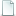## Point Set Registration: Coherent Point Drift A. Myronenko and X. Song10.1.1.250.5954.pdf 6.61MB
Type: Paper
Tags:

Bibtex:
```@ARTICLE{5432191,
author={A. Myronenko and X. Song},
journal={IEEE Transactions on Pattern Analysis and Machine Intelligence},
title={Point Set Registration: Coherent Point Drift},
year={2010},
volume={32},
number={12},
pages={2262-2275},
abstract={Point set registration is a key component in many computer vision tasks. The goal of point set registration is to assign correspondences between two sets of points and to recover the transformation that maps one point set to the other. Multiple factors, including an unknown nonrigid spatial transformation, large dimensionality of point set, noise, and outliers, make the point set registration a challenging problem. We introduce a probabilistic method, called the Coherent Point Drift (CPD) algorithm, for both rigid and nonrigid point set registration. We consider the alignment of two point sets as a probability density estimation problem. We fit the Gaussian mixture model (GMM) centroids (representing the first point set) to the data (the second point set) by maximizing the likelihood. We force the GMM centroids to move coherently as a group to preserve the topological structure of the point sets. In the rigid case, we impose the coherence constraint by reparameterization of GMM centroid locations with rigid parameters and derive a closed form solution of the maximization step of the EM algorithm in arbitrary dimensions. In the nonrigid case, we impose the coherence constraint by regularizing the displacement field and using the variational calculus to derive the optimal transformation. We also introduce a fast algorithm that reduces the method computation complexity to linear. We test the CPD algorithm for both rigid and nonrigid transformations in the presence of noise, outliers, and missing points, where CPD shows accurate results and outperforms current state-of-the-art methods.},
keywords={Gaussian processes;computational complexity;computer vision;estimation theory;expectation-maximisation algorithm;image registration;probability;CPD algorithm;EM algorithm;GMM centroid locations;GMM centroids;Gaussian mixture model;coherence constraint;coherent point drift;computation complexity;computer vision;nonrigid transformations;optimal transformation;point set registration;probabilistic method;probability density estimation;reparameterization;topological structure;unknown nonrigid spatial transformation;Coherent Point Drift (CPD);EM algorithm.;Gaussian mixture model (GMM);Registration;alignment;coherence;correspondence;matching;nonrigid;point sets;regularization;rigid},
doi={10.1109/TPAMI.2010.46},
ISSN={0162-8828},
month={Dec},}
```

Send Feedback